# Trinomials of the formwhereMost trinomials do not factor with integer values. However, it is always satisfying to find trinomials that do. Factoring helps us to solve equations efficiently, to graph relations easily and to develop mathematical arguments. All trinomials on this page do factor nicely.

A trinomial wherecan be tricky to factor. On this page, we think strategically through each problem to find the solution.

An alternative technique is to use a an algorithm called  factoring by decomposition.

A third technique is a grid method: Factor Trinomial using a Grid Method

Here are some examples of thinking through a trinomial strategically.

Example 1: No constant termThe highest common factor of these two terms is.Example 2: There is a common factor between all three termsFirst we notice that 2 divides all three terms. Let’s factor it out:Now, within the brackets, we have a trinomial withand so we simply find a factor pair that multiplies to 4 and adds to -5. I’m thinking -4 and -1 will do it!Example 3: Whereis a prime number.Now, when we lay out the brackets, the only integer pair that multiplies to the leading term isand. So we begin with that:Next, we need to choose between factor pairs 9, 1 (or 1, 9) and 3, 3 to make the product c = 9. We also note that they both have to be negative. (why?) We guess and check until it works out.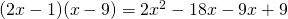nopeagain, nolovely. Done!

A prime number as a coefficient ofreduces the number of guesses we need to perform.Example 4: Whereis a prime number (or 1)Now, when we lay out the brackets, the only integer pair that multiplies to the constant termisand. So we begin with that:Now we need to choose between factor pairs 4, 1 (or 1, 4) or 2,2 for the leading coefficient. Let’s try them out:No, this give +3x in the middle.Nice, this does it.

After only two guesses, we reach our solution:Example 5: Where the coefficientis negative.Our first step here is to factor out the negative, along with any common numerical factor.We then focus our attention on what is inside the bracket. In this example, the coefficient ofis, a prime number.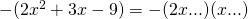Trying out factor pairs for 9 in the last place: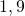thenthen: we find that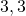works to give the linear term, therefore:Example 6: Where neithernoris a prime numberIn this example we might need to do extensive scribbling in the margin. We must also remember that one of the primary reasons we factor is for speed and efficiency. If we do not find a factorization soon enough, chances are high (when you’re not on this page) your trinomial does not factor nicely. If we are trying to factor in order to be able to solve an equation or to graph, and if it is taking too long, we use a different technique for solution – either ‘completing the square’ or ‘the quadratic formula’. These are taught in a different course.

On our side for this example is the fact that it is a ‘+, +’ trinomial. Our search is limited to just positive values.

Factor pairs for 10 are: 1, 10; also 2, 5 and vice versa for each.

Factor pairs for 9 are: 1, 9 and vice versa; and  3,3.

Let’s begin our checking out the options: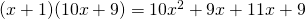No, this givesin the middle…. we really only have to examine the middle terms.No, this givesin the middle.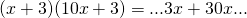Great! We have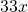in the middle. So we have our solution:Practice with this applet or use the kuta software worksheet linked below. Be sure to multiply out mentally (or on paper) before you click ‘check’.

Kuta Software Worksheet

Done here! Go back to the Factors and Products Menu.